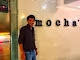Bangalore, India
I am an Oracle Certified Professional working in SAP Labs. Everything written on my blog has been tested on my local environment, Please test before implementing or running in production. You can contact me at amit.rath0708@gmail.com.

## Tuesday, October 15, 2013

### Concept of ROWNUM, Why ROWNUM=2 gives "no rows selected

ROWNUM is a psuedocolumn , its value is not predefined in a table. A number is assigned in the form of 1,2,3,...N to every row returned by a query when its executed, that number is called as ROWNUM.  Its generated dynamically.

Example :- Consider a table having 5 rows. PFB output of below select queries :-

1. SQL> select * from amit;

ID NAME
---------- ------------------------------------
1
2
3
4
5

2. SQL> select * from amit where rownum=1;

ID NAME
---------- ------------------------------------
1

3. SQL> select * from amit where rownum<3;

ID NAME
---------- ------------------------------------
1
2

4. SQL> select * from amit where rownum=2;

no rows selected

5. SQL> select * from amit where rownum>2;

no rows selected

We see that when we use Rownum in Where clause then it gives output only for rownum<3 and Rownum=1 . But when we we use rownum=2 and rownum>2 it gives output as "no rown selected".

Reason for this behaviour of rownum is that its value is not predefined. when a select query fetch some output then a number is assingned in the form of 1,2,3...N to all rows fetched by query.First selected row is always assigned as rownum=1.

Rownum value is incremented after a query passes the where clause.

So when we use rownum=1 or rownum<3 in where clause then where condition is matched as rownum=1 at that time value of rownum is 1 so it passes the where condition and gives us the output. IF rownum < 3 has been used in where clause then it gives us output for rownum=1 as it passes where clasuse, now rownum value incremented and becomes 2 again it passes where clause (rownum<3 ). Now  rownum value incremented and becomes 3, when rownum value becomes 3 then it fails the where clause(rownum<3) and gives us only two rows as output.

But when We have rownum=2 or rownum>2 then numbers is assigned to the output as 1,2,3..N , when first row's rownum which is 1 is matched with rownum=2 or rownum>2 then at this time rownum has value as 1 and it fails the where condition(rownum=2 or rownum>2) criteria on first attempt and gives us output as "no rows selected"

We have to remember this statement that "Rownum is not preassigned in table"